## Main

The uncertainty relation was first proposed by Heisenberg2 in 1927 as a limitation of simultaneous measurements of canonically conjugate variables owing to the back-action of the measurement: the measurement of the position Qof the electron with the error ε(Q), or ‘the mean error’, induces the disturbance η(P), or ‘the discontinuous change’, of the momentum P so that they always satisfy the relation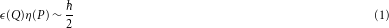where is Planck’s constant divided by 2π (here, we use /2 for consistency with modern treatments). In a mathematical derivation of the above relation from the commutation relation Q PP Q=i, Heisenberg2 used the reciprocal relation σ(Q)σ(P)≥/2 for standard deviations σ(Q), σ(P) of position and momentum, which was proved shortly afterwards in ref. 3 for arbitrary wavefunctions. This relation was generalized to arbitrary pairs of observables A, B by Robertson4 as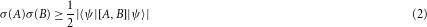in any states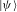with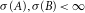. Here, [A,B]represents the commutator [A,B]=A BB A and the standard deviation is defined as σ(A)2=〈ψ|A2|ψ〉−〈ψ|A|ψ2. Note that a positive definite covariance term can be added to the right-hand side of equation (2), if squared, as discussed by Schrödinger5. For our experimental setting, this term vanishes. Robertson’s relation (equation (2)) for standard deviations has been confirmed by many different experiments. In a single-slit diffraction experiment15 the uncertainty relation, as expressed in equation (2), has been confirmed. A trade-off relation appears in squeezing coherent states of radiation fields16, and many experimental demonstrations have been carried out17.

Robertson’s relation (equation (2)) has a mathematical basis, but has no immediate implications for limitations on measurements. This relation is naturally understood as limitations on state preparation or limitations on prediction from the past. On the other hand, the proof of the reciprocal relation for the error ε(A) of an A measurement and the disturbance η(B) on observable B caused by the measurement, in a general form of Heisenberg’s error–disturbance relation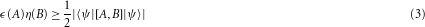is not straightforward, as Heisenberg’s proof2 used an unsupported assumption on the state just after the measurement12, despite successful justifications for the Heisenberg-type relation for unbiased joint measurements8,9,10. Recently, rigorous and general theoretical treatments of quantum measurements have revealed the failure of Heisenberg’s relation (equation (1)), and derived a new universally valid relation11,12,13,14 given by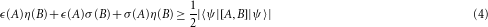Here, the error ε(A) is defined as the root mean squared (r.m.s.) of the difference between the output operator OAactually measured and the observable A to be measured, whereas the disturbance η(B) is defined as the r.m.s. of the change in observable B during the measurement11,13 (see Methods, ‘Universally valid uncertainty relation’, for details). The additional second and third terms result mathematically from non-commutativity between B (A) and the error (disturbance) operator (Equation (235) in ref. 13) and imply a new accuracy limit ε(A)≥(1/2)|〈ψ|[A,B]|ψ〉|σ(B)−1 for non-disturbing (η(B)=0) measurements and a new disturbance limit η(B)≥(1/2)|〈ψ|[A,B]|ψ〉|σ(A)−1 for noise-free (ε(A)=0) measurements, instead of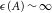or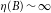as derived from the Heisenberg-type relation (equation (3)). Note that the above relations (equations (3) and (4)) are based on the state-dependent formulation of error and disturbance for arbitrary observables. For the position–momentum case, there are different approaches based on the state-independent formulation18,19,20, where the lower bound for error–disturbance inequality depends strongly on the measures used.

We have experimentally tested the universally valid error–disturbance relation (equation (4)) for neutron-spin measurements21. We set A and B as the x and y components of the neutron 1/2 spin. (For simplicity, /2 is omitted for each spin component.) The error ε(A) and the disturbance η(B) are defined for a measuring apparatus called M1, so that apparatus M1 measures the observable A=σx with error ε(A)and disturbs the observable B=σy with disturbance η(B)during the measurement, where σx and σy denote the Pauli matrices. To control the error ε(A) and the disturbance η(B), apparatus M1 is designed to actually carry out the projective measurement of OA=σϕ=σxcosϕ+σysinϕinstead of exactly measuring A=σxby detuning the azimuthal angle ϕ of σϕ between 0 and π/2, which is an experimentally controlled parameter, so that ε(A) and η(B)are determined as a function of ϕ (see Methods for details). As the output operator OA and the observable A to be measured are not simultaneously measurable, their difference is not a directly detectable quantity, and neither is the change of the observable B. On this ground, the notions of the error ε(A) and the disturbance η(B) have been often claimed to be experimentally inaccessible19,22. To overcome this difficulty, we use the method proposed in ref. 13 to determine the error ε(A) and the disturbance η(B) from experimentally available data (see Methods for details). A different method for the experimental demonstration of the same relation (equation (4)), which exploits the weak-measurement technique used for measuring momentum transfer in ref. 23, was recently proposed in ref. 24.

In our experiment, a neutron beam passes a preparation stage of the initial state. The error ε(A) is determined by the data from the apparatus M1, and the disturbance η(B) is determined by the data from another apparatus called M2, which carries out the projective measurement of B on the state just after the M1 measurement. Thus, the experiment is based on the successive projective measurements of two non-commuting observables OA in M1 and B in M2 as depicted in Fig. 1. The apparatus M1 has two possible outcomes, that is +1 and −1, corresponding to measurement operators Eϕ(±1)=(1/2)(I±σϕ). The apparatus M2 also yields either +1 or −1, corresponding to measurement operators Ey(±1)=(1/2)(I±σy). Thus, the successive measurements carried out by M1 and M2 finally result in four intensities denoted as (+ +), (+ −), (− +) and (− −). The set-up of the neutron spin experiment is depicted in Fig. 2. In the actual experiment the spin analysers select only one spin component. Hence, the four possible outcomes are recorded not at the same time, as in an ideal projective measurement, but one after the other. As seen from equations (7) and (8), error ε(A) and disturbance η(B) for the state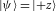are obtained by making the successive measurements of OA and B on the state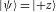as well as the auxiliary states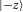,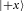and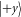.

The experiment was carried out at the research reactor facility TRIGA Mark II of the Vienna University of Technology. The monochromatic neutron beam with a mean wavelength of 1.96 Å propagates in the +y direction. The beam is approximately 99% polarized crossing a bent Co–Ti supermirror array (polarizer)25. Two analysing supermirrors (analysers) are adjusted to higher incident angles so that the second-order harmonics in the incident beam are suppressed. The final intensity was about 90 neutrons s−1 at a beam cross-section of 10 (vertical) × 5 (horizontal) mm2. A 3He monitor detector is used for normalization to correct statistical fluctuations evoked by the reactor power. A BF3 detector with high efficiency (more than 99%) is used for the experiment. To avoid unwanted depolarization, a static guide field pointing in the +z direction with a strength of about 10 Gauss permeates rectangular Helmholtz coils. In addition, the guide field induces Larmor precession, which, together with four appropriately placed d.c. spin rotator coils, enables state preparation and projective measurements of OA in M1 and B in M2 (see Methods for details).

To test the universally valid uncertainty relation stated in equation (4), the standard deviations σ(A) and σ(B), the error ε(A) and the disturbance η(B) are determined. The measurements of the standard deviations σ(A) and σ(B) are carried out by M1 and M2 separately, whereas error ε(A) and disturbance η(B) are determined by successive projective measurements using M1 and M2.

Typical experimental data sets, for miscellaneous detuning angles ϕ, are depicted in Fig. 3. The resulting values of ε(A) and η(B), together with the theoretical predictions ε(A)=2sin(ϕ/2) and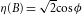(Methods), are plotted as a function of the detuning parameter ϕ in Fig. 4. The trade-off relation of ε(A) and η(B) is in good agreement with theory: when one observable is measured more precisely, the other is more disturbed. The calculated expectation values are normalized owing to the limiting efficiency of the entire apparatus (96%), so that expectation values are bounded between ±1 (Methods). Errors are calculated using error propagation from the standard deviation of the count rates and considering inaccuracies of the Larmor precession angles (1.5°): the latter stem mainly from inhomogeneity of the guide field along the beam.

From the terms obtained above (error ε(A), disturbance η(B), standard deviations σ(A) and σ(B)), the Heisenberg error–disturbance product ε(A)η(B)and the universally valid expression ε(A)η(B)+ε(A)σ(B)+σ(A)η(B), that is, the left-hand side of (equation (4)), are plotted as a function of the detuned azimuthal angle ϕ in Fig. 5a. This figure illustrates the fact that the Heisenberg product is always below the calculated limit, and that the universally valid expression is always larger than the limit in the scanned range of ϕ.Figure 5: Experimentally determined values of the universally valid uncertainty relation.

The invalidity of the naive Heisenberg limit for projective measurements of bounded observables, as in the present experiment, has been discussed in ref. 14. In fact, the projective measurements of A and B achieve ε(A)=0 and η(B)=0, respectively, whereas we have 0≤η(B),ε(A)≤2 for any measurement. Thus, at least some region around these points (ϕ=0 and ϕ=π/2) is expected to exhibit the violation of equation (3). Moreover, owing to our choice of observables and the initial state, we keep the Heisenberg product ε(A)η(B) below the limit throughout the entire range of ϕ.

Although the error is experimentally controlled by a single parameter here, it is easily extendable for uncontrolled and fluctuating parameters. Here, we concentrate on the situation where the full trade-off relation between error ε(A) and disturbance η(B) occurs. It is worth noting that the mean value of the observable A is correctly reproduced for any detuning angle ϕ, that is, 〈+z|OA|+z〉=〈+z|A|+z〉, so that the projective measurement of OA reproduces the correct probability distribution of A, whereas we can detect the non-zero r.m.s. error ε(A) for ϕ≠0. What has been shown by the uncertainty principle is the existence of an unavoidable trade-off between measurement accuracy and disturbance, but this principle has eluded a satisfactory quantitative description for a long time. Our demonstration is the first evidence for the validity of the new relation (equation (4)) proposed as a universally valid error–disturbance relation11,12,13,14; moreover the failure of the old relation (equation (3)) is also illustrated. Our result demonstrates that the new relation solves a long-standing problem of describing the relation between measurement accuracy and disturbance, and sheds light on fundamental limitations of quantum measurements, for instance on the debate of the standard quantum limit for monitoring free-mass position26,27,28.

## Methods

### Universally valid uncertainty relation.

Any measuring apparatus M is, in principle, modelled by the unitary operator U describing the time evolution of the composite system of the measured object S and the probe system P during the measuring interaction and the meter observable M of P actually measured after the measuring interaction13. If the initial states of the object and the apparatus are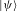and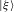, respectively, the r.m.s. error ε(A) of Mfor measuring an observable A of Sand the r.m.s. disturbance η(B) of M caused on an observable B of S are defined as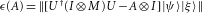where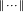denotes the norm of the state vector and I stands for the identity operator. Then, it is mathematically proved11,13 that equation (4) holds for any unitary operator U for S+P, observable M of P and state vectorsof S andof P. Suppose that the apparatus M has a family {Mm} of measurement operators29. This means that the measuring apparatus M has possible outcomes m with probability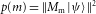and the state of the object Safter the measurement with outcome m is. In this case, the r.m.s. error and r.m.s. disturbance are given by14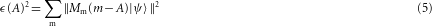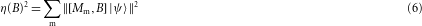If {Mm} consists of mutually orthogonal projections, the measurement is called a projective measurement. In this case, equation (5) can be simplified as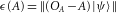by the Pythagorean theorem, where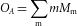is called the output operator.

### Error and disturbance in spin measurements: theoretical determination.

In the experiment, we test the universally valid uncertainty relation (equation (4)) for observables A=σx and B=σy, where the initial stateis |+z〉and the measuring apparatus M=M1 is considered to carry out the projective measurement of OA=σϕ=cosϕ σx+sinϕ σy. Thus, the apparatus M1 is described by measurement operators Eϕ(+1)=(1+σϕ)/2 and Eϕ(−1)=(1−σϕ)/2 with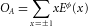. From equations (5) and (6), we have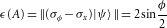### Experimental determination of error and disturbance.

In the experiment, we determine ε(A) and η(B) from statistically available data obtained by successive neutron spin measurements. According to the former theoretical analysis (refs. 13, p. 387), the error ε(A)is determined by mean values of OA in three different states as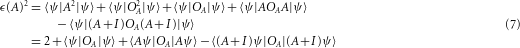where we have used the following abbreviations:and. As apparatus M1 carries out the projective measurement of OA, to determine ε(A) for the basic initial statewe need only to measure the intensities from apparatus M1 in the three auxiliary incident states of M1 corresponding to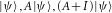. The expectation values expressed in equation (7) are calculated from the measured intensities, depicted in Fig. 3, using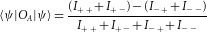To detect the disturbance on B caused by apparatus M1, apparatus M2 carries out the projective measurement of B in the state just after the M1 measurement. The modified output operators of apparatus M2 for the initial state of M1 are given by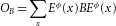and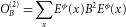, which describe the mean and the second moment of the M2 measurement for the initial state of M1. Then, from Eqs. (189) and (227) of ref. 13 the disturbance η(B)is also determined by mean values of OB in three different states as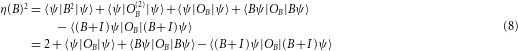where the similar notations |B ψ〉 and |(B+I)ψ〉 are used as before and the expectation values are given by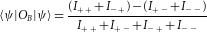Thus η(B) is determined in the same manner as ε(A). By the relations,,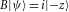,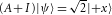, and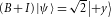, where we set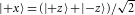and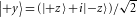, the required states in equations (7) and (8) are generated by spinor rotations in the experiment (Fig. 2); the normalization factors are confirmed experimentally in spin-rotation measurement. Note that for a general observable X the statecan be prepared with success probability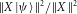by applying a ‘completely positive operation’30. Thus, the statesand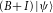can be prepared in principle without knowing what the stateis.

### State preparation and successive neutron spin measurements.

By using a supermirror polarizer, the incoming monochromatic neutron beam (Δλ/λ0.02) is highly polarized (99%) in the +z direction. The state of the neutrons is controlled basically by four d.c. coil spin-turners (Fig. 2). The first d.c. coil (d.c.-1) produces a magnetic field in the x direction (Bx), which can be used to rotate the inital polarization vector about the x axis. By further exploiting Larmor precession about the +z axis, induced by the static guide field present throughout the entire set-up, and varying the position of d.c.-1, arbitrary initial states can be produced at the end of the preparation stage (up to an irrelevant phase factor). In our particular configuration the required states are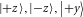and. For the generation of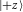d.c.-1 is switched off, whereas the spin is flipped by d.c.-1 forpreparation. The state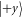is generated by applying a π/2 rotation around the x axis and thestate is produced by further moving the position of d.c.-1 by one-quarter of the Larmor rotation period. The projective measurement of observable OA is realized by similar mechanisms: the prepared state rotates about the z axis owing to Larmor precession. Hence, by properly placing the d.c.-2 coil, the spin component to be measured can be projected towards the +z direction, where it is reflected by the supermirror analyser-1. After passing through this first analyser in the +z state, d.c.-3 produces the eigenstate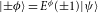of σϕ so that the apparatus M1 makes the projective measurement of OAto obtain the mean values of OA in equation (7). In the same manner, apparatus M2 makes the measurement of observable B on the eigenstate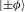, which detects the disturbance on the observable B. Hence we obtain the mean values of OB in equation (8) from apparatus M2. The combination of the projective measurements of σϕ and σy gives four count rates at the neutron detector in the downstream of the beam. Note that the measurement made by apparatus M2 is the error-free B measurement on the state just after the measurement carried out by M1.